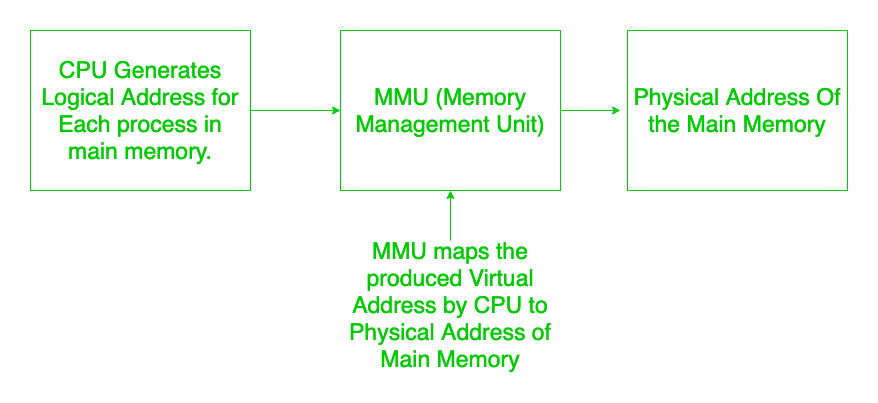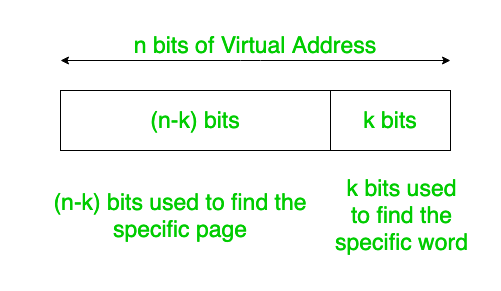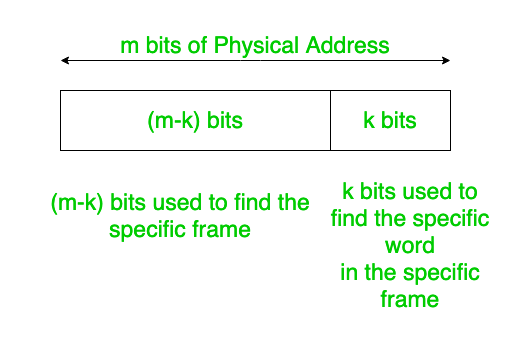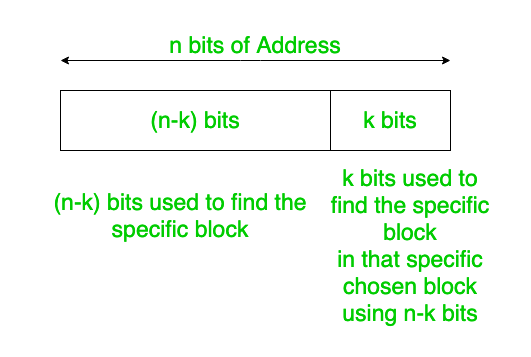# Concept Of Address Split in OS

It is generated by the CPU.The meaning of the previous sentence is that our systems are designed so that CPU utilisation should be maximum and thus to use the CPU properly we sort to use multiprogramming. Due to Multiprogramming it is not that only complete process is loaded into the main memory, instead many different number of processes may be present in the main memory . And when each of this processes are loaded to CPU the CPU thinks that only process in present in the main memory. Thus, it produces Addresses relative to 0, it means that CPU starts treating the process as if it is loaded to memory location 0 in the main memory. Thus, for every process (during context switch) CPU would produce address relative to 0.

Now each of the process is divided into pages of equal length and in return each page consists of fixed number of words.

To keep track of which pages are loaded where in Main Memory we use a Page Table. The number of page table entries is equal to number of pages. Just like array index the indices represent the Page number and respective content would hold the location where the Page is actually Loaded in the Main Memory. Some times even the page we need might not be present in the Page Table thus Page Miss occurs and in that case we need to Fetch the page from the secondary memory.

As explained above that CPU generates addresses of all the processes in the main memory relative to zero i.e., CPU produces address starting from 0.Now the addresses generated by are no way real because if those addresses were valid then all processes would begin from zero and rest spaces in Main Memory would be memory. Thus the addresses generated by CPU should be some way mapped to real (physical address) of the Main Memory. This is done by MMU (Memory Management unit).Now we usually divide the Main Memory in to Frames of equal sizes.And in return each of the Frame consists of equal number of words.

Important Point to be noted is that Page Size = Frame Size.

Note:
The smallest addressable unit in memory is called word. It means the smallest unit which can specifically identified using address bits.

The Address Space of Virtual Address is divided into 2 parts: Page Number, and Page OFFset.

Similarly, the Address Space of Physical Address is divided into 2 parts: Frame Number, and Frame OFFset.

Let Virtual Address has n bits and Physical Address has m bits.

`Page Size = Frame Size = k bits `

(as page size = Frame size as mentioned above).

`Number of Pages = 2(n-k) pages``Number of Frames = 2(m-k) Frames`And, number of word in each page/frame = 2(k) pages.

Thus in both the addressing schemes last k bits are reserved to find the particular word.Left n-k bits are used to find the Page number from all the set of page numbers and in case of frame the rest m-k bits are used to find out the particular frame from all the set of frame numbers.

In simple terms I can explain here that of n bits in Virtual Address n-k bits are find the particular page number in page table and m-k bits of m bits of Physical Address are used to find the particular frame and after find the particular frame we need to go to particular word containing the required data (since word is the smallest addressable unit) so the k bits are used to find that particular.

Trick:
Consider a n bit address space then if k bits are used to define number of blocks in that address space the rest n-k bits are used to find a specific block along that chosen block using the k bits. This would be clear from the below block diagram.This same concept can be extended also in Computer Networks.

Note: